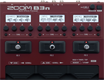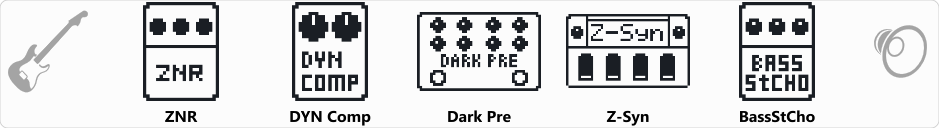# Factory : HysterSyn

Discussion in 'Zoom B3n' started by Tonelib, May 20, 2018.

1. Factory : HysterSynDevice: Zoom B3n
Firmware: 2.00

Name on device: HysterSyn
Optimized for: Phones/Speaker

Effects chain:A famous song by MUSE is the inspiration for this setting.

Effect: "ZNR" (Dynamics), active - "yes"
"Detect" = GTRIN
"Depth" = 95
"Threshold" = 55
"Decay" = 20

Effect: "DYN Comp" (Dynamics), active - "yes"
"Sense" = 7
"Attack" = Slow
"Tone" = 50
"Volume" = 35

Effect: "Dark Pre" (Overdrive / Distortion), active - "yes"
"Bass" = 70
"Lo-Mid" = 35
"Hi-Mid" = 75
"Treble" = 50
"Blend" = 100
"Gain" = 83
"Volume" = 18
"Boost" = LO+HI

Effect: "Z-Syn" (Sfx), active - "yes"
"Frequency" = 7
"Range" = 18
"Decay" = 90
"Resonance" = 10
"Wave" = SAW
"Tone" = 5
"Balance" = 30
"Volume" = 85
"Frequency" = 3
"Range" = 16
"Decay" = 75
"Resonance" = 18
"Wave" = SAW
"Tone" = 4
"Balance" = 60
"Volume" = 70
"Parameter set" = A

Effect: "BassStCho" (Modulation), active - "yes"
"Depth" = 35
"Rate" = 10
"LoCut" = 400 Hz
"Mix" = 50

Patch Volume: 120